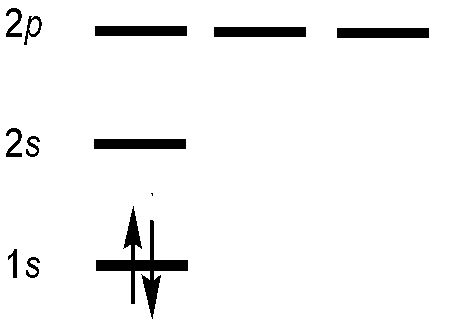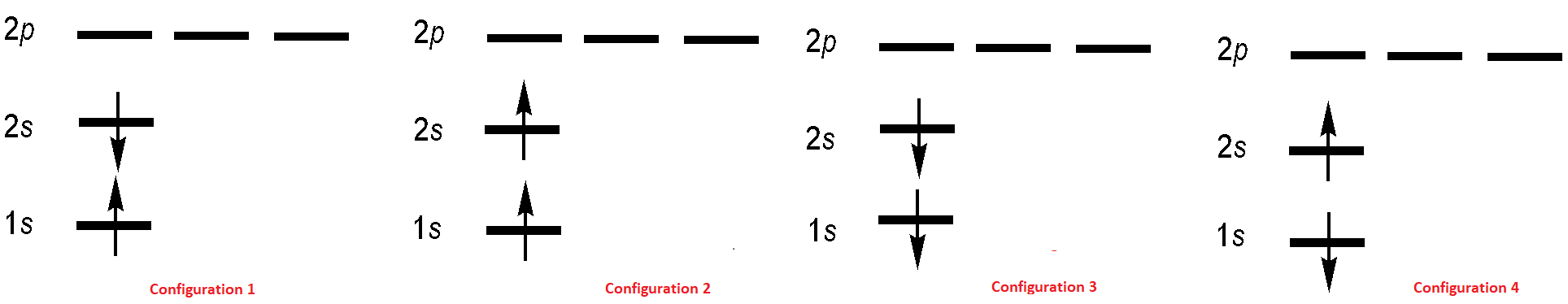# 8.6: Antisymmetric Wavefunctions can be Represented by Slater Determinants

Let’s try to construct an antisymmetric function that describes the two electrons in the ground state of helium. Blindly following the first statement of the Pauli Exclusion Principle, then each electron in a multi-electron atom must be described by a different spin-orbital. For the ground-state helium atom, this gives a $$1s^22s^02p^0$$ configuration (Figure $$\PageIndex{1}$$).Figure $$\PageIndex{1}$$: Electron configuration for ground state of the helium atom.

We try constructing a simple product wavefunction for helium using two different spin-orbitals. Both have the 1s spatial component, but one has spin function $$\alpha$$ and the other has spin function $$\beta$$ so the product wavefunction matches the form of the ground state electron configuration for He, $$1s^2$$.

$| \psi (\mathbf{r}_1, \mathbf{r}_2 ) \rangle = \varphi _{1s\alpha} (\mathbf{r}_1) \varphi _{1s\beta} ( \mathbf{r}_2) \label {8.6.1}$

After permutation of the electrons, this becomes

$| \psi ( \mathbf{r}_2,\mathbf{r}_1 ) \rangle = \varphi _{1s\alpha} ( \mathbf{r}_2) \varphi _{1s\beta} (\mathbf{r}_1) \label {8.6.2}$

which is different from the starting function since $$\varphi _{1s\alpha}$$ and $$\varphi _{1s\beta}$$ are different spin-orbital functions. Hence, the simple product wavefunction in Equation \ref{8.6.1} does not satisfy the indistinguishability requirement since an antisymmetric function must produce the same function multiplied by (–1) after permutation of two electrons, and that is not the case here.We must try something else. To avoid getting a totally different function when we permute the electrons, we can make a linear combination of functions. A very simple way of taking a linear combination involves making a new function by simply adding or subtracting functions. The function that is created by subtracting the right-hand side of Equation $$\ref{8.6.2}$$ from the right-hand side of Equation $$\ref{8.6.1}$$ has the desired antisymmetric behavior. The constant on the right-hand side accounts for the fact that the total wavefunction must be normalized.$| \psi (\mathbf{r}_1, \mathbf{r}_2) \rangle = \dfrac {1}{\sqrt {2}} [ \varphi _{1s\alpha}(\mathbf{r}_1) \varphi _{1s\beta}( \mathbf{r}_2) - \varphi _{1s\alpha}( \mathbf{r}_2) \varphi _{1s\beta}(\mathbf{r}_1)] \label{8.6.3}$​We can introduce a shorthand notation for the arbitrary spin-orbital

$\varphi_{i\alpha}(\mathbf{r_j}) = \varphi_i \alpha(j)$

or

$\varphi_{i\beta}(\mathbf{r_j}) = \varphi_i \beta(j)$

as determined by the $$m_s$$ quantum number. This wavefunction in Equation \ref{8.6.3} can be decomposed into spatial and spin components:

$| \psi (\mathbf{r}_1, \mathbf{r}_2) \rangle = \dfrac {1}{\sqrt {2}} \underbrace{[ \varphi _{1s}(1) \varphi _{1s}(2)]}_{\text{spatial component}} \underbrace{[ \alpha(1) \beta( 2) - \alpha( 2) \beta(1)]}_{\text{spin component}} \label{8.6.3B}$

Exercise $$\PageIndex{1}$$

Show that the linear combination in Equation $$\ref{8.6.3}$$ is antisymmetric with respect to permutation of the two electrons. (Hint: replace the minus sign with a plus sign (i.e. take the positive linear combination of the same two functions) and show that the resultant linear combination is symmetric).

The first excited state of He is $$1s^12s^12p^0$$ and we can envision four microstates for this configuration (Figure $$\PageIndex{2}$$) that also must satisfy indistinguishability requirement just like the ground state.Figure $$\PageIndex{2}$$: Electron configurations for first-excited state of the helium atom.

These correspond to the following two-electron wavefunctions:

\begin{align} | \psi_1 (\mathbf{r}_1, \mathbf{r}_2) \rangle &= \dfrac {1}2 \underbrace{[ \varphi _{1s}(1) \varphi _{2s}(2)+\varphi _{1s}(2) \varphi _{2s}(1)]}_{\text{spatial component}} \underbrace{[ \alpha(1) \beta( 2) - \alpha( 2) \beta(1)]}_{\text{spin component}} \label{8.6.3C1} \\[4pt] | \psi_2 (\mathbf{r}_1, \mathbf{r}_2) \rangle &= \dfrac {1}{\sqrt {2}} \underbrace{[ \varphi _{1s}(1) \varphi _{2s}(2) - \varphi _{1s}(2) \varphi _{2s}(1)]}_{\text{spatial component}} \underbrace{[ \alpha(1) \alpha( 2)]}_{\text{spin component}} \label{8.6.3C2} \\[4pt] | \psi_3 (\mathbf{r}_1, \mathbf{r}_2) \rangle &= \dfrac {1}{\sqrt {2}} \underbrace{[ \varphi _{1s}(1) \varphi _{2s}(2) - \varphi _{1s}(2) \varphi _{2s}(1)]}_{\text{spatial component}} \underbrace{[ \alpha(1) \beta( 2) + \alpha( 2) \beta(1)]}_{\text{spin component}} \label{8.6.3C3} \\[4pt] | \psi_4 (\mathbf{r}_1, \mathbf{r}_2) \rangle &= \dfrac {1}2 \underbrace{[ \varphi _{1s}(1) \varphi _{2s}(2) - \varphi _{1s}(2) \varphi _{2s}(1)]}_{\text{spatial component}} \underbrace{[ \beta(1) \beta( 2)]}_{\text{spin component}} \label{8.6.3C4} \end{align}

All four wavefunctions are antisymmetric as required for fermionic wavefunctions. Functions $$| \psi_1 \rangle$$ and $$| \psi_2 \rangle$$ correspond to the two electrons both having spin up or both having spin down. For many electrons, this ad hoc construction procedure would obviously become unwieldy. However, there is an elegant way to construct an antisymmetric wavefunction for a system of N identical particles.

## Determinantal Wavefunctions

A linear combination that describes an appropriately antisymmetrized multi-electron wavefunction for any desired orbital configuration is easy to construct for a two-electron system. However, interesting chemical systems usually contain more than two electrons. For these multi-electron systems a relatively simple scheme for constructing an antisymmetric wavefunction from a product of one-electron functions is to write the wavefunction in the form of a determinant. John Slater introduced this idea so the determinant is called a Slater determinant.

John C. Slater introduced the determinants in 1929 as a means of ensuring the antisymmetry of a wavefunction, however the determinantal wavefunction first appeared three years earlier independently in Heisenberg's and Dirac's papers.

The Slater determinant for the two-electron wavefunction of helium is

$| \psi (\mathbf{r}_1, \mathbf{r}_2) \rangle = \dfrac {1}{\sqrt {2}} \begin {vmatrix} \varphi _{1s} (1) \alpha (1) & \varphi _{1s} (1) \beta (1) \\ \varphi _{1s} (2) \alpha (2) & \varphi _{1s} (2) \beta (2) \end {vmatrix} \label {8.6.4}$

A shorthand notation for the determinant in Equation $$\ref{8.6.4}$$ is then

$| \psi (\mathbf{r}_1 , \mathbf{r}_2) \rangle = 2^{-\frac {1}{2}} Det | \varphi _{1s\alpha} (\mathbf{r}_1) \varphi _{1s\beta} ( \mathbf{r}_2) | \label {8.6.5}$

The determinant is written so the electron coordinate changes in going from one row to the next, and the spin orbital changes in going from one column to the next. The advantage of having this recipe is clear if you try to construct an antisymmetric wavefunction that describes the orbital configuration for uranium! Note that the normalization constant is $$(N!)^{-\frac {1}{2}}$$ for $$N$$ electrons.

The generalized Slater determinant for a multi-electrom atom with $$N$$ electrons is then

$\psi(\mathbf{r}_1, \mathbf{r}_2, \ldots, \mathbf{r}_N)=\dfrac{1}{\sqrt{N!}} \left| \begin{matrix} \varphi_1(\mathbf{r}_1) & \varphi_2(\mathbf{r}_1) & \cdots & \varphi_N(\mathbf{r}_1) \\ \varphi_1(\mathbf{r}_2) & \varphi_2(\mathbf{r}_2) & \cdots & \varphi_N(\mathbf{r}_2) \\ \vdots & \vdots & \ddots & \vdots \\ \varphi_1(\mathbf{r}_N) & \varphi_2(\mathbf{r}_N) & \cdots & \varphi_N(\mathbf{r}_N) \end{matrix} \right| \label{5.6.96}$

Now that we have seen how acceptable multi-electron wavefunctions can be constructed, it is time to revisit the “guide” statement of conceptual understanding with which we began our deeper consideration of electron indistinguishability and the Pauli Exclusion Principle. What does a multi-electron wavefunction constructed by taking specific linear combinations of product wavefunctions mean for our physical picture of the electrons in multi-electron atoms? Overall, the antisymmetrized product function describes the configuration (the orbitals, regions of electron density) for the multi-electron atom. Because of the requirement that electrons be indistinguishable, we can’t visualize specific electrons assigned to specific spin-orbitals. Instead, we construct functions that allow each electron’s probability distribution to be dispersed across each spin-orbital. The total charge density described by any one spin-orbital cannot exceed one electron’s worth of charge, and each electron in the system is contributing a portion of that charge density.

The four wavefunctions outlined above for first-excited state of the helium atom can be expressed as the following Slater Determinantal wavefunctions

$| \psi_1 (\mathbf{r}_1, \mathbf{r}_2) \rangle = \dfrac {1}{\sqrt {2}} \begin {vmatrix} \varphi _{1s} (1) \alpha (1) & \varphi _{1s} (1) \alpha(1) \\ \varphi _{2s} (1) \beta(1) & \varphi _{2s} (2) \beta (2) \end {vmatrix} \label {8.6.10A}$

$| \psi_2 (\mathbf{r}_1, \mathbf{r}_2) \rangle = \dfrac {1}{\sqrt {2}} \begin {vmatrix} \varphi _{1s} (1) \alpha (1) & \varphi _{1s} (1) \beta (1) \\ \varphi _{2s} (1) \alpha (1) & \varphi _{2s} (2) \alpha(2) \end {vmatrix} \label {8.6.10B}$

$| \psi_3 (\mathbf{r}_1, \mathbf{r}_2) \rangle = \dfrac {1}{\sqrt {2}} \begin {vmatrix} \varphi _{1s} (1) \beta(1) & \varphi _{1s} (1) \beta (1) \\ \varphi _{2s} (1) \beta(1) & \varphi _{2s} (2) \beta (2) \end {vmatrix} \label {8.6.10C}$

$| \psi_4 (\mathbf{r}_1, \mathbf{r}_2) \rangle = \dfrac {1}{\sqrt {2}} \begin {vmatrix} \varphi _{1s} (1) \beta(1) & \varphi _{1s} (1) \beta (1) \\ \varphi _{2s} (1) \alpha (1) & \varphi _{2s} (2) \alpha(2) \end {vmatrix} \label {8.6.10D}$

Exercise $$\PageIndex{3}$$

Show that the determinant wavefunction (Equation \ref{8.6.4} is the same as the form for the helium wavefunction that is given in Equation \ref{8.6.3}.

Exercise $$\PageIndex{4}$$

Expand the Slater determinant in Equation $$\ref{8.6.4}$$ for the He atom.

Exercise $$\PageIndex{5}$$

Write and expand the Slater determinant for the Li atom.

Exercise $$\PageIndex{7}$$

Write the Slater determinant for the carbon atom. If you expanded this determinant, how many terms would be in the linear combination of functions?

Exercise $$\PageIndex{8}$$

Write the Slater determinant for the $$1s^12s^1$$ excited state orbital configuration of the helium atom.

Exercise $$\PageIndex{9}$$

Critique the energy level diagram and shorthand electron configuration notation from the perspective of the indistinguishability criterion. Can you imagine a way to represent the wavefunction expressed as a Slater determinant in a schematic or shorthand notation that more accurately represents the electrons? (This is not a solved problem!)